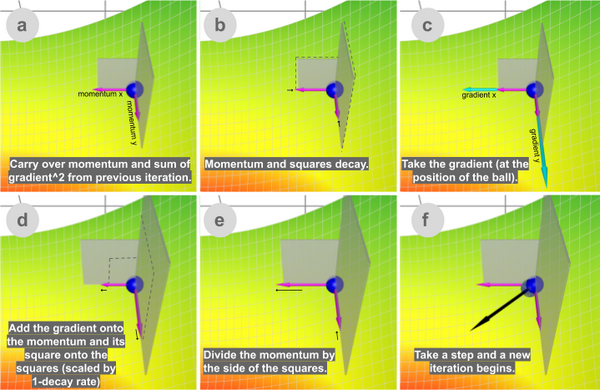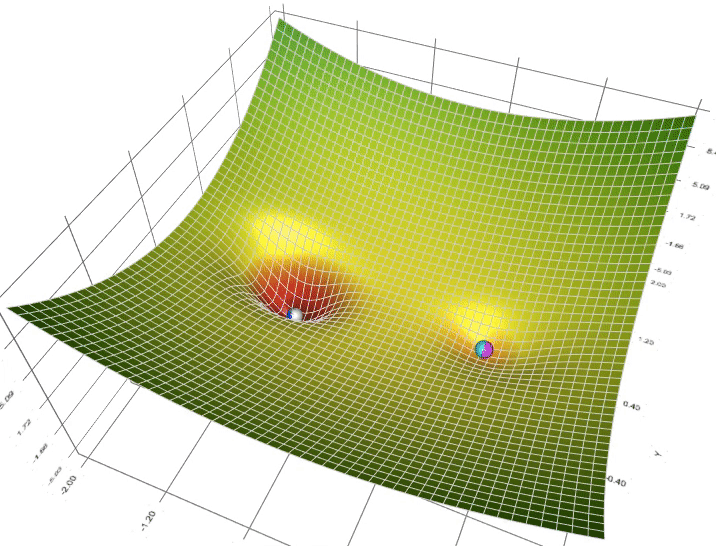delta = - learning_rate * gradient

theta += delta

Theta 是一些需要优化的参数(例如，神经网络中神经元与神经元之间连接的权重，线性回归特征的系数，等等)。 在机器学习优化设置中可能有成千上万个这样的 thetasDelta 是算法中每次迭代后 theta 的变化量; 希望随着每次这样的变化，theta 逐渐接近最优值。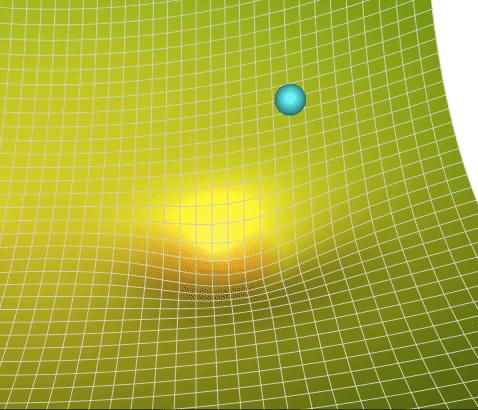## 动量（Momentum）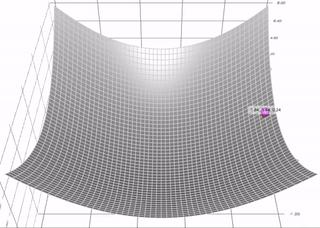delta = - learning_rate * gradient + previous_delta * decay_rate (方程1)

theta += delta (方程2)

delta = -learning_rate * sum_of_gradient (方程4)

theta += delta (方程5)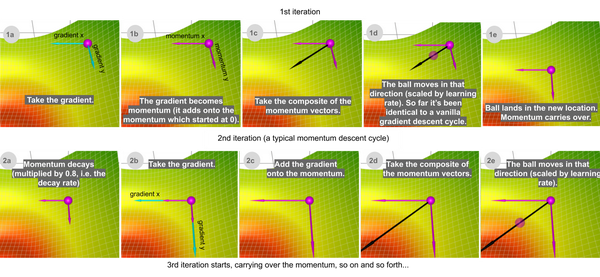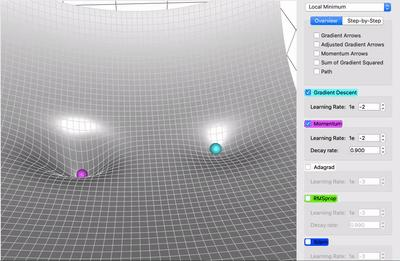1. 动量移动得更快(因为它积累的所有动量)
2. 动量有机会逃脱局部极小值(因为动量可能推动它脱离局部极小值)。 同样，我们将在后面看到，它也将更好地通过高原区

theta += delta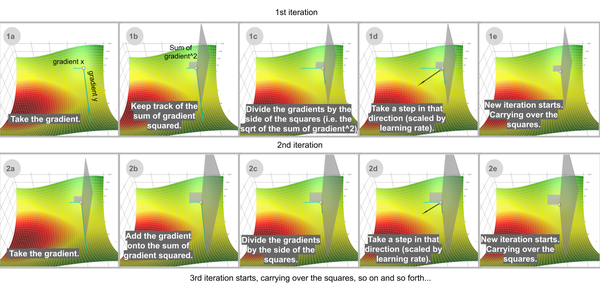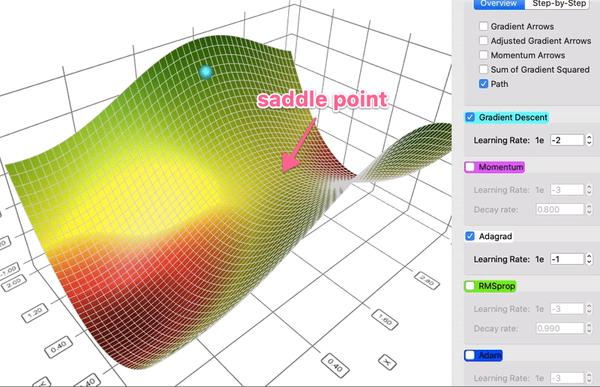## RMSProp

theta += delta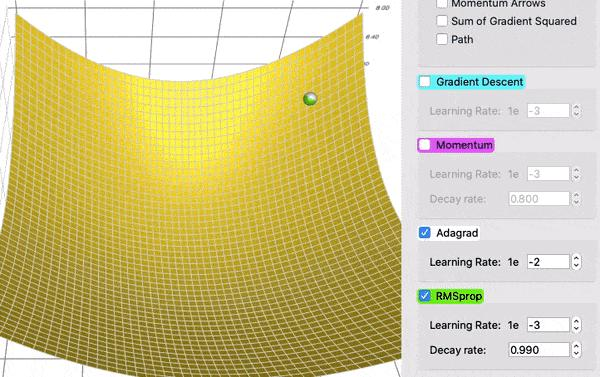theta += delta

Beta1是一阶矩梯度之和(动量之和)的衰减率，通常设置为0.9。 Beta2是二阶矩梯度平方和的衰减率，通常设置为0.999。